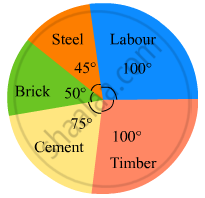Share

# The Pie-chart Given in the Following Represents the Expenditure on Different Items in Constructing a Flat in Delhi. If the Expenditure Incurred on Cement is Rs 112500, Find the Following: - Mathematics

Course
ConceptIntroduction of Circle Graph Or Pie Chart

#### Question

The pie-chart given in the following represents the expenditure on different items in constructing a flat in Delhi. If the expenditure incurred on cement is Rs 112500, find the following:(i) Total cost of the flat.
(ii) Expenditure incurred on labour.

#### Solution

(i) Expenditure incurred on cement  =

$\frac{\text{ Central angle of the corresponding sector } \times\text{ Total cost }}{360^\circ}$
Total cost of the flat = $\frac{360^\circ \times 112500}{75^\circ} =\text{ Rs }540000$
(ii) Expenditure incurred on labour = $\frac{\text{ Central angle of labour sector }\times\text{ Total cost }}{360^\circ}$$= \frac{100^\circ \times 540000}{360^\circ} =\text{ Rs }150000$
Is there an error in this question or solution?

#### APPEARS IN

RD Sharma Solution for Mathematics for Class 8 by R D Sharma (2019-2020 Session) (2017 to Current)
Chapter 25: Data Handling-III (Pictorial Representation of Data as Pie Charts or Circle Graphs)
Ex. 25.2 | Q: 1 | Page no. 21

#### Video TutorialsVIEW ALL 

Solution The Pie-chart Given in the Following Represents the Expenditure on Different Items in Constructing a Flat in Delhi. If the Expenditure Incurred on Cement is Rs 112500, Find the Following: Concept: Introduction of Circle Graph Or Pie Chart.
S